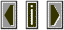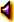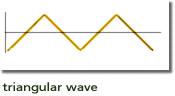Triangle waveA wave form in the shape of a triangle which contains only odd harmonics.    the harmonics above the fundamental dont have as much energy as those of a pulse wave.triangleThe amplitude of a specific harmonic (relative to the amplitude of the fundamental) is square of the inverse of its harmonic number:   1/n**2 where n is the partial number. Thus the fifth harmonic has 1/25th the amplitude of the fundamental.